# Trigonometric polynomial

(diff) ← Older revision | Latest revision (diff) | Newer revision → (diff)

finite trigonometric sum

An expression of the form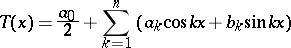with real coefficients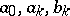,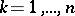; the numberis called the order of the trigonometric polynomial (provided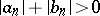). A trigonometric polynomial can be written in complex form: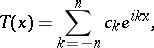where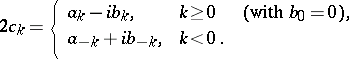Trigonometric polynomials are an important tool in the approximation of functions.